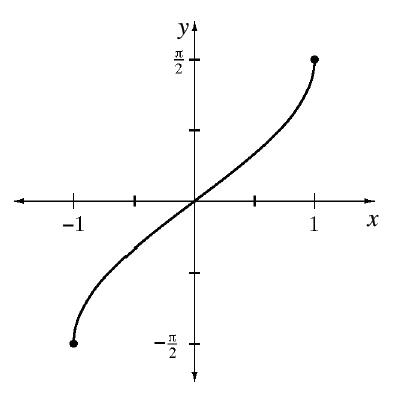### Home > PC3 > Chapter 10 > Lesson 10.1.3 > Problem10-66

10-66.

Graph two complete cycles of each of the following trigonometric functions.

1. $f(x)=$$-4\cos(\frac{1}{5}(x-2))$

This is a cosine function with an amplitude of $4$, reflected vertically, shifted right $2$ units, and with a period of $2π/(1/5)$.

1. $g(x)=$$-5\sin^{-1}(x)+1$

$y = \sin^{−1}(x)$